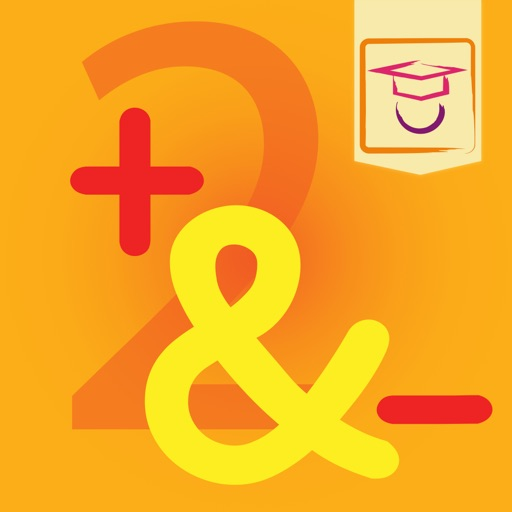## Can you already add and subtract with numbers up to 20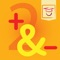# Plus & Minus 2 - Magiwise

by Magiwise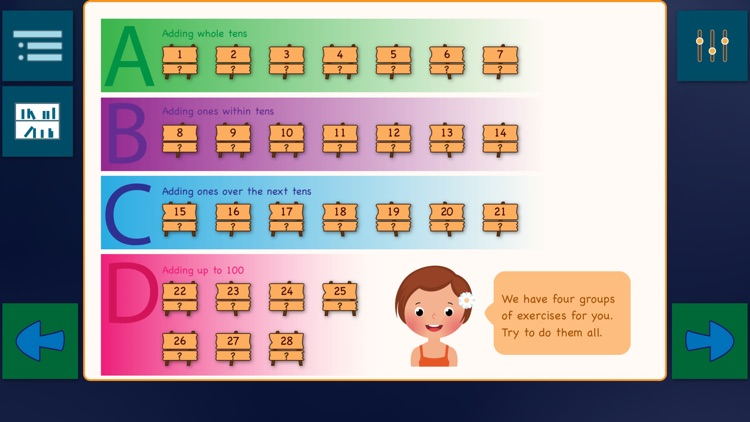Can you already add and subtract with numbers up to 20? Then it's time to move on. In this app, you practice additions with numbers up to 100. Calculating with tens is the first part, then in 3 steps more difficult situations occur. After you have done all the exercises, you can perform mental calculations with plus sums up to the number 100.### App Details

Version
6.2
Rating
NA
Size
106Mb
Genre
Education Family Puzzle
Last updated
December 4, 2022
Release date
December 17, 2019

### App Screenshots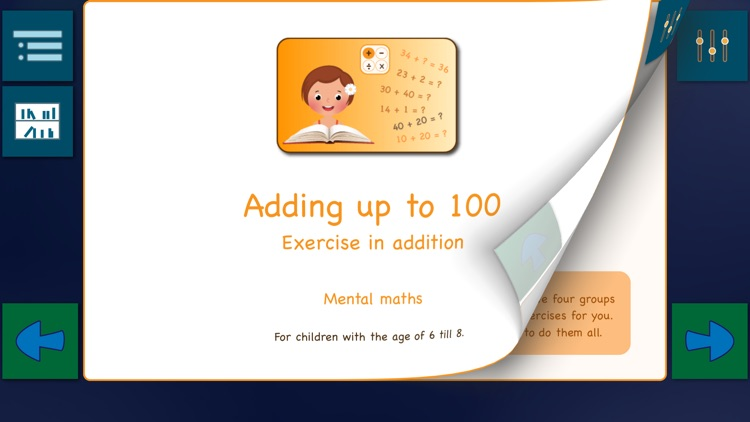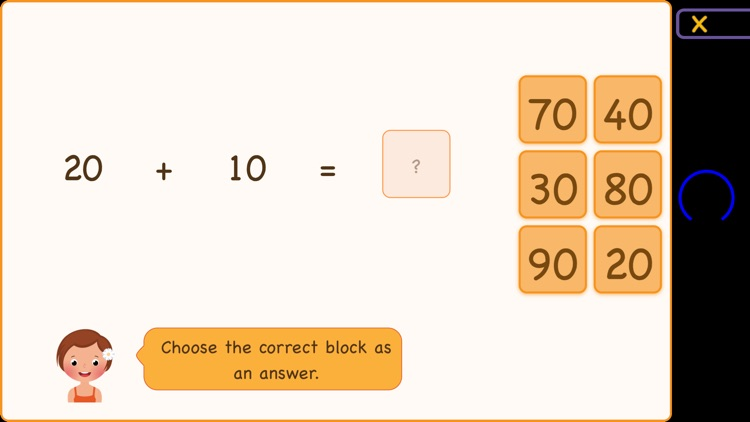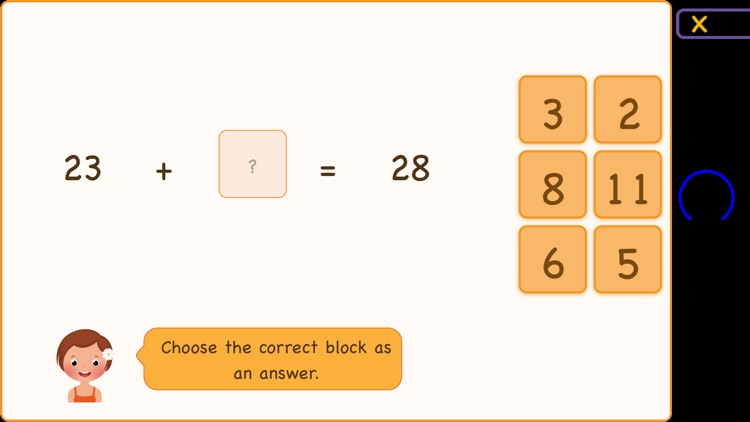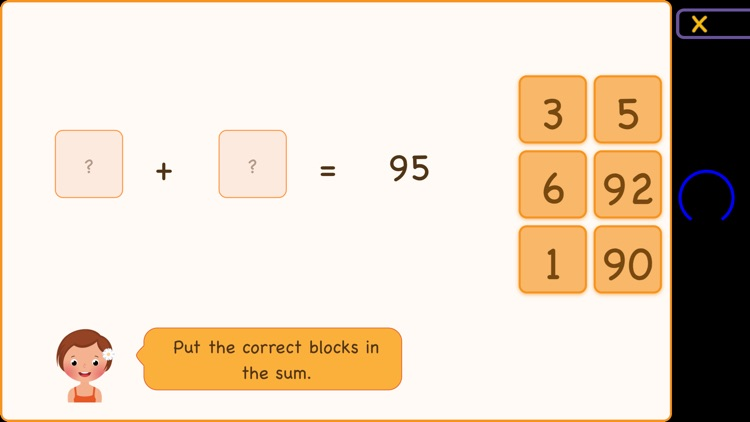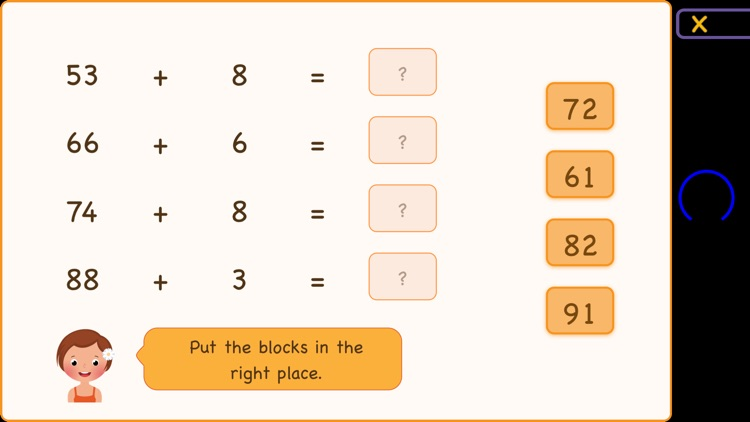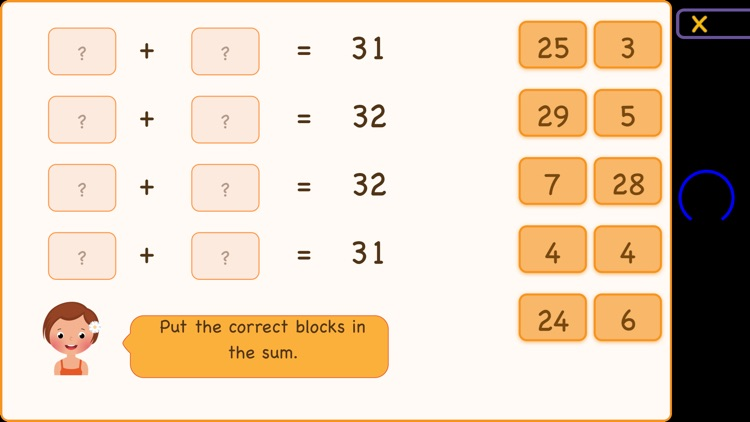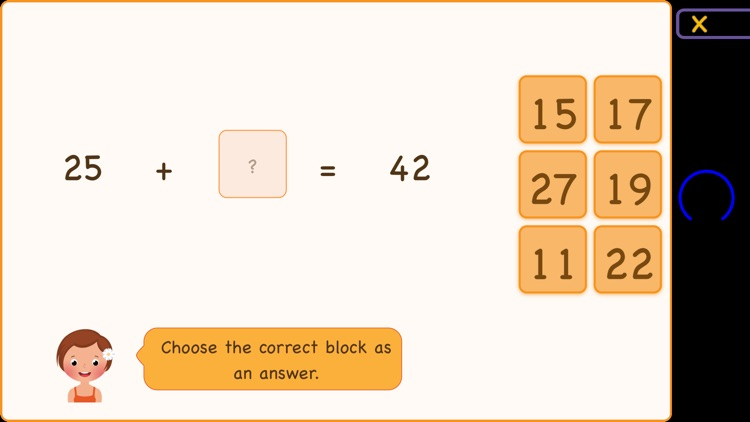### App Store Description

Can you already add and subtract with numbers up to 20? Then it's time to move on. In this app, you practice additions with numbers up to 100. Calculating with tens is the first part, then in 3 steps more difficult situations occur. After you have done all the exercises, you can perform mental calculations with plus sums up to the number 100.

Learn to add up to 100 in four steps
The exercises start easily, but you will also meet considerable challenges to solve. We divided the exercises for you into the following four groups:
- Addition of tens (e.g.: 20 + 10)
- Addition within tens (e.g.: 20 + 3)
- Addition over the next ten. (e.g.: 22 + 11)
- Addition with numbers below 100 (eg: 27 + 34)

Every group contains several exercises, for example:

Solve a sum one by one.
During the first exercise in each group, the app shows a sum on the screen. You have to put the correct answer behind the '='-sign. Later you also get an exercise in which various sums are shown on a screen at the same time.

You are listening to the sum.
In this exercise, you listen to the voice that reads the sum. Then you have to type in the answer. In this way, you learn to add without making choices from possible solutions on the screen.